# 词汇嵌入:编码词汇语义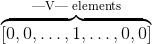• The mathematician ran to the store.
• The physicist ran to the store.
• The mathematician solved the open problem.

• The physicist solved the open problem.

• 我们看到数学家和物理学家在句子中有着相同的作用.它们之间有一个语义关系.
• 我们已经看到数学家在这个新的没看过的的句子中扮演着同样的角色, 就像我们现在看到的物理学家一样.

## Getting Dense Word Embeddings(密集字嵌入)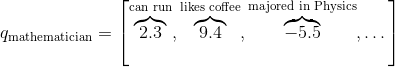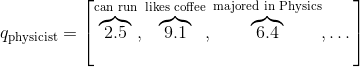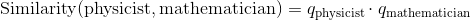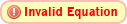## Word Embeddings in Pytorch（Pytorch中的单词嵌入）

# 作者: Robert Guthrie

import torch
import torch.nn as nn
import torch.nn.functional as F
import torch.optim as optim

torch.manual_seed(1)

word_to_ix = {"hello": 0, "world": 1}
embeds = nn.Embedding(2, 5)  # 2 单词, 5 维嵌入
lookup_tensor = torch.LongTensor([word_to_ix["hello"]])
print(hello_embed)


## 例子: N-Gram 语言模型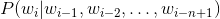是句子中第i个单词.

CONTEXT_SIZE = 2
EMBEDDING_DIM = 10
# 我们将使用 Shakespeare Sonnet 2
test_sentence = """When forty winters shall besiege thy brow,
And dig deep trenches in thy beauty's field,
Thy youth's proud livery so gazed on now,
Will be a totter'd weed of small worth held:
Then being asked, where all thy beauty lies,
Where all the treasure of thy lusty days;
To say, within thine own deep sunken eyes,
Were an all-eating shame, and thriftless praise.
How much more praise deserv'd thy beauty's use,
If thou couldst answer 'This fair child of mine
Shall sum my count, and make my old excuse,'
Proving his beauty by succession thine!
This were to be new made when thou art old,
And see thy blood warm when thou feel'st it cold.""".split()
# 我们应该对输入进行标记,但是我们将忽略它
# 建造一系列元组.  每个元组 ([ word_i-2, word_i-1 ], 都是目标单词)
trigrams = [([test_sentence[i], test_sentence[i + 1]], test_sentence[i + 2])
for i in range(len(test_sentence) - 2)]
# 输出前 3, 为了让你看到他的各式
print(trigrams[:3])

vocab = set(test_sentence)
word_to_ix = {word: i for i, word in enumerate(vocab)}

class NGramLanguageModeler(nn.Module):

def __init__(self, vocab_size, embedding_dim, context_size):
super(NGramLanguageModeler, self).__init__()
self.embeddings = nn.Embedding(vocab_size, embedding_dim)
self.linear1 = nn.Linear(context_size * embedding_dim, 128)
self.linear2 = nn.Linear(128, vocab_size)

def forward(self, inputs):
embeds = self.embeddings(inputs).view((1, -1))
out = F.relu(self.linear1(embeds))
out = self.linear2(out)
log_probs = F.log_softmax(out, dim=1)
return log_probs

losses = []
loss_function = nn.NLLLoss()
model = NGramLanguageModeler(len(vocab), EMBEDDING_DIM, CONTEXT_SIZE)
optimizer = optim.SGD(model.parameters(), lr=0.001)

for epoch in range(10):
total_loss = torch.Tensor()
for context, target in trigrams:

# 步骤 1\. 准备好进入模型的数据 (例如将单词转换成整数索引,并将其封装在变量中)
context_idxs = [word_to_ix[w] for w in context]

# 步骤 2\. 回调 *积累* 梯度. 在进入一个实例前,需要将之前的实力梯度置零

# 步骤 3\. 运行反向传播,得到单词的概率分布
log_probs = model(context_var)

# 步骤 4\. 计算损失函数. (再次注意, Torch需要将目标单词封装在变量中)
torch.LongTensor([word_to_ix[target]])))

# 步骤 5\. 反向传播并更新梯度
loss.backward()
optimizer.step()

total_loss += loss.data
losses.append(total_loss)
print(losses)  # 在训练集中每次迭代损失都会减小!


## Exercise: Computing Word Embeddings: Continuous Bag-of-Words(练习: 计算单词嵌入: 连续单词包)

CBOW模型如下所示.给定一个目标单词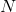代表单词每一遍的滑窗距,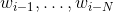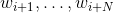, 将所有上下文词统称为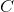,CBOW试图去最小化如下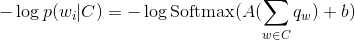• 想好你需要定义的系数.
• 确保你知道每一步操作后的构造. 如果想要重构请使用 .view().
CONTEXT_SIZE = 2  # 左右各2个单词
raw_text = """We are about to study the idea of a computational process.
Computational processes are abstract beings that inhabit computers.
As they evolve, processes manipulate other abstract things called data.
The evolution of a process is directed by a pattern of rules
called a program. People create programs to direct processes. In effect,
we conjure the spirits of the computer with our spells.""".split()

# 通过从 raw_text 得到一组单词, 进行去重操作
vocab = set(raw_text)
vocab_size = len(vocab)

word_to_ix = {word: i for i, word in enumerate(vocab)}
data = []
for i in range(2, len(raw_text) - 2):
context = [raw_text[i - 2], raw_text[i - 1],
raw_text[i + 1], raw_text[i + 2]]
target = raw_text[i]
data.append((context, target))
print(data[:5])

class CBOW(nn.Module):

def __init__(self):
pass

def forward(self, inputs):
pass

# 创建模型并且训练. 这里有一些函数可以在使用模型之前帮助你准备数据

def make_context_vector(context, word_to_ix):
idxs = [word_to_ix[w] for w in context]
tensor = torch.LongTensor(idxs)